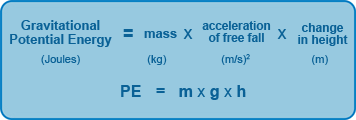# What is the gravitational potential energy of a  15 kg object on a shelf  6/5 m  high?

Aug 26, 2016

$176.6 J$

#### Explanation:

The answer can be obtained by using the equation below:Let's identify the known and unknown variables:

$\textcolor{b l u e}{\text{Knowns:}}$
- Mass
- Change in height
- I am going to assume that the acceleration of free fall is $9.81 \frac{m}{s} ^ \left(2\right)$

$\textcolor{m a \ge n t a}{\text{Unknowns:}}$
- Gravitational potential energy

Our known variables have good units, so all we have to do is plug the given values into the equation and solve for the gravitational potential energy like this:

$\text{gravitational potential energy} =$

$15 k g \times 9.81 \frac{m}{s} ^ \left(2\right) \times \left(\frac{6}{5}\right) m$ $= 176.6 J$ or $176.6 \frac{k g \times {m}^{2}}{{s}^{2}}$

Note: